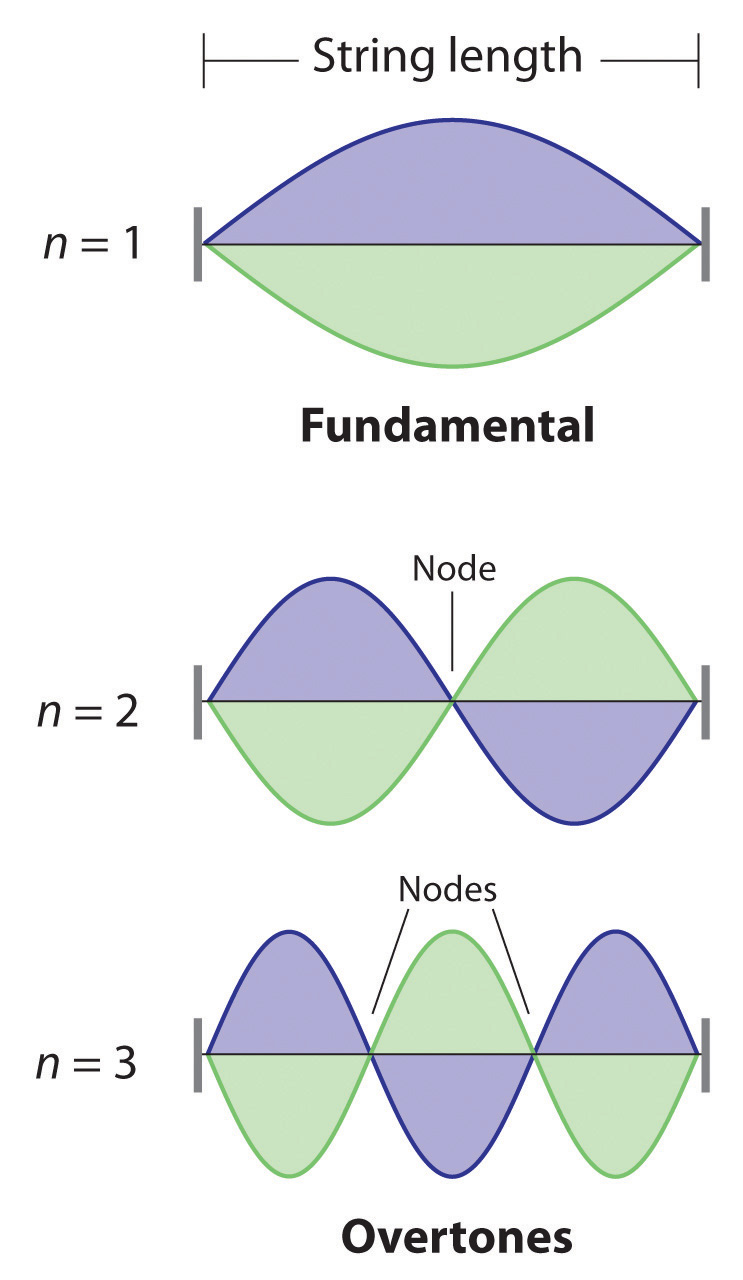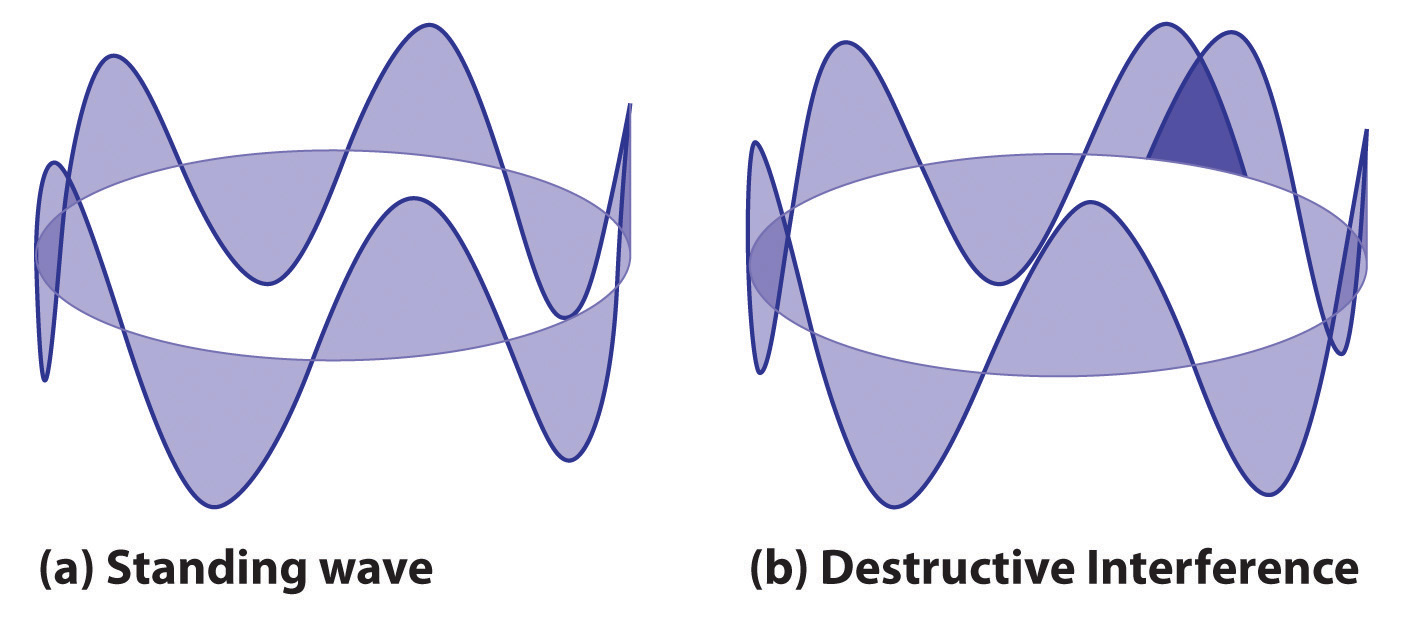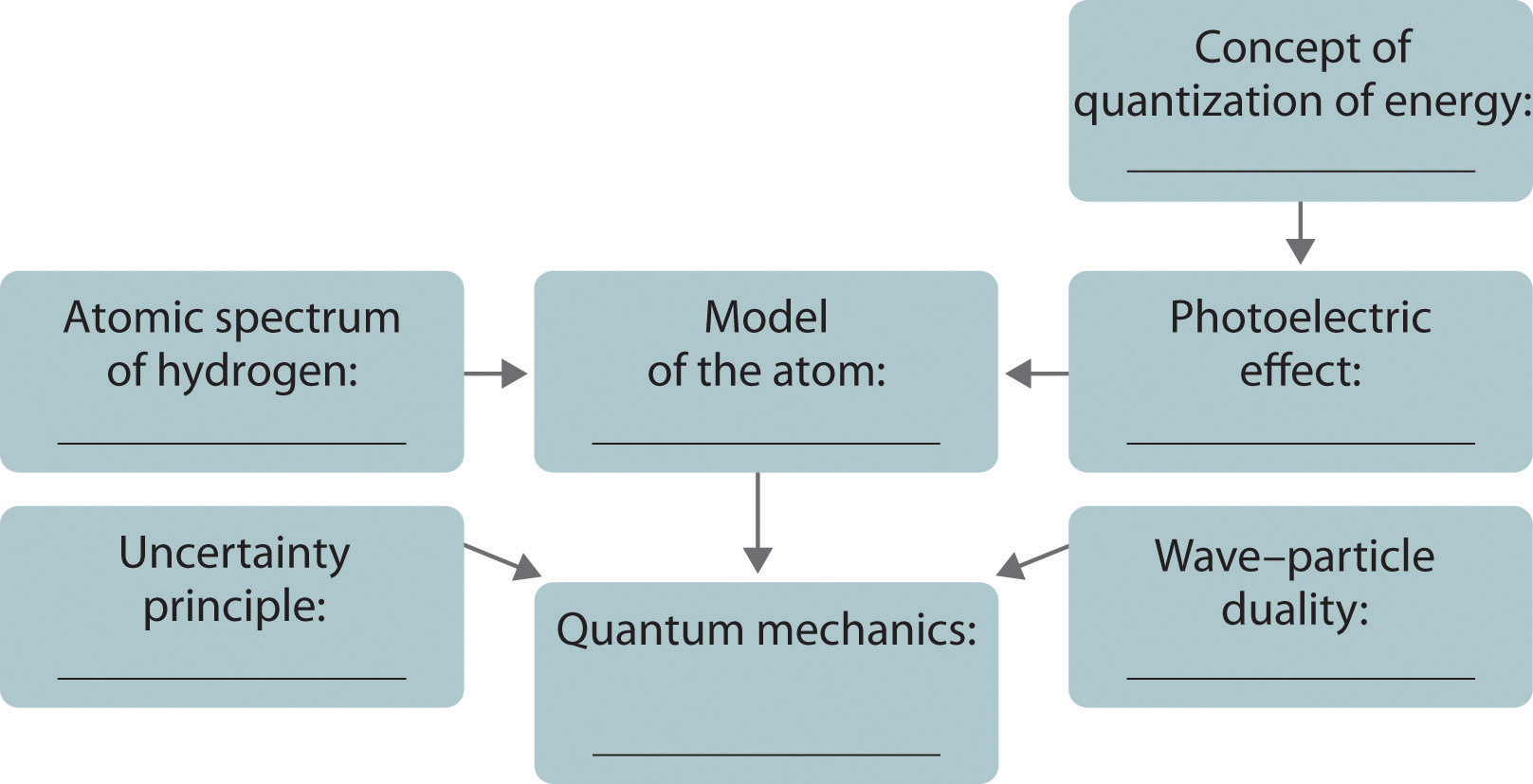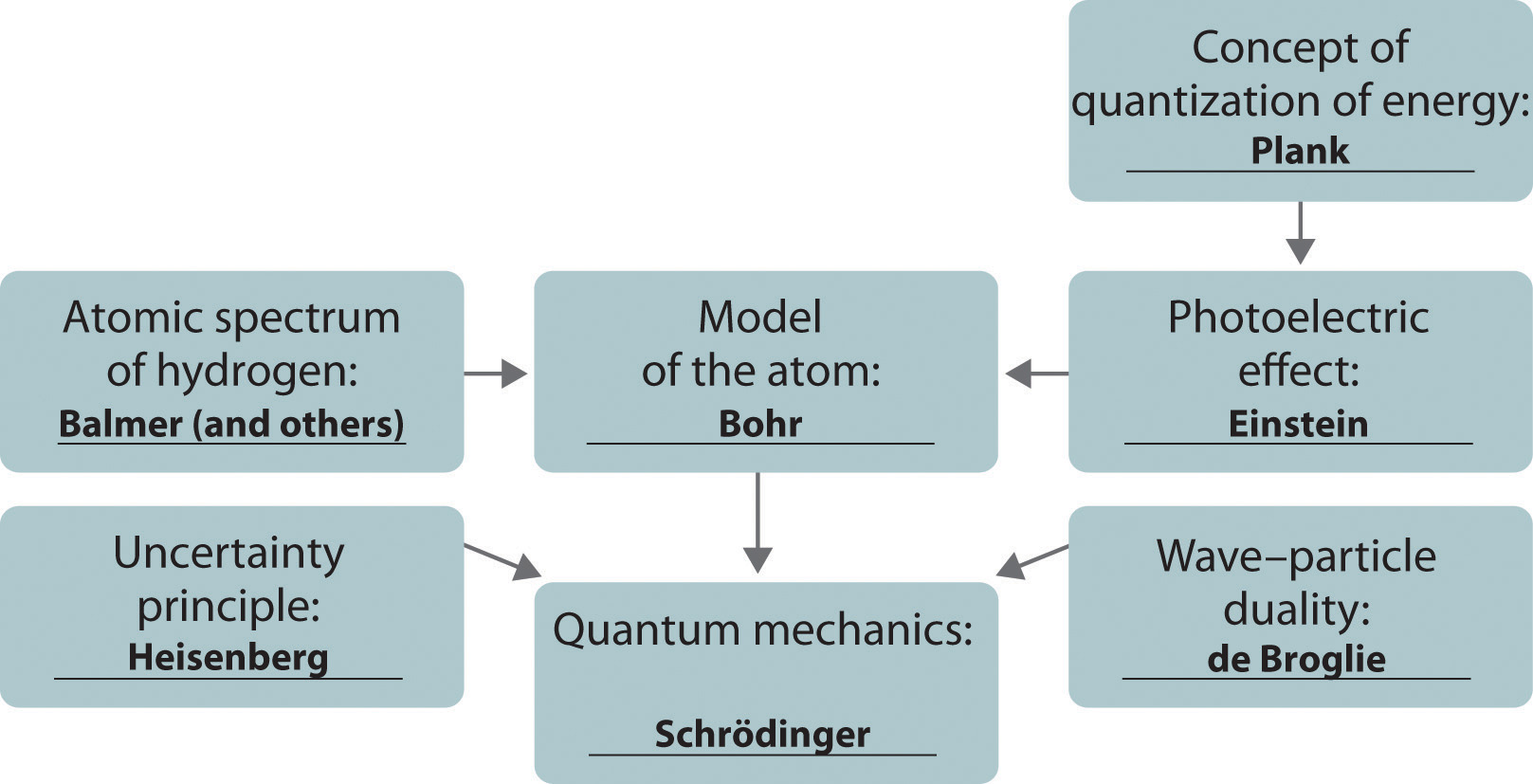This is “The Relationship between Energy and Mass”, section 6.4 from the book Principles of General Chemistry (v. 1.0). For details on it (including licensing), click here.

Has this book helped you? Consider passing it on:
Creative Commons supports free culture from music to education. Their licenses helped make this book available to you.
DonorsChoose.org helps people like you help teachers fund their classroom projects, from art supplies to books to calculators.

## 6.4 The Relationship between Energy and Mass

### Learning Objective

1. To understand the wave–particle duality of matter.

Einstein’s photons of light were individual packets of energy having many of the characteristics of particles. Recall that the collision of an electron (a particle) with a sufficiently energetic photon can eject a photoelectron from the surface of a metal. Any excess energy is transferred to the electron and is converted to the kinetic energy of the ejected electron. Einstein’s hypothesis that energy is concentrated in localized bundles, however, was in sharp contrast to the classical notion that energy is spread out uniformly in a wave. We now describe Einstein’s theory of the relationship between energy and mass, a theory that others built on to develop our current model of the atom.

## The Wave Character of Matter

Einstein initially assumed that photons had zero mass, which made them a peculiar sort of particle indeed. In 1905, however, he published his special theory of relativity, which related energy and mass according to the following equation:

Equation 6.13

$E = h ν = h c λ = m c 2$

According to this theory, a photon of wavelength λ and frequency ν has a nonzero mass, which is given as follows:

Equation 6.14

$m = E c 2 = h ν c 2 = h λ c$

That is, light, which had always been regarded as a wave, also has properties typical of particles, a condition known as wave–particle dualityA principle that matter and energy have properties typical of both waves and particles.. Depending on conditions, light could be viewed as either a wave or a particle.

In 1922, the American physicist Arthur Compton (1892–1962) reported the results of experiments involving the collision of x-rays and electrons that supported the particle nature of light. At about the same time, a young French physics student, Louis de Broglie (1892–1972), began to wonder whether the converse was true: Could particles exhibit the properties of waves? In his PhD dissertation submitted to the Sorbonne in 1924, de Broglie proposed that a particle such as an electron could be described by a wave whose wavelength is given by

Equation 6.15

$λ = h m v$

where h is Planck’s constant, m is the mass of the particle, and v is the velocity of the particle. This revolutionary idea was quickly confirmed by American physicists Clinton Davisson (1881–1958) and Lester Germer (1896–1971), who showed that beams of electrons, regarded as particles, were diffracted by a sodium chloride crystal in the same manner as x-rays, which were regarded as waves. It was proven experimentally that electrons do exhibit the properties of waves. For his work, de Broglie received the Nobel Prize in Physics in 1929.

If particles exhibit the properties of waves, why had no one observed them before? The answer lies in the numerator of de Broglie’s equation, which is an extremely small number. As you will calculate in Example 4, Planck’s constant (6.63 × 10−34 J·s) is so small that the wavelength of a particle with a large mass is too short (less than the diameter of an atomic nucleus) to be noticeable.

### Example 4

Calculate the wavelength of a baseball, which has a mass of 149 g and a speed of 100 mi/h.

Given: mass and speed of object

Strategy:

A Convert the speed of the baseball to the appropriate SI units: meters per second.

B Substitute values into Equation 6.15 and solve for the wavelength.

Solution:

The wavelength of a particle is given by λ = h/mv. We know that m = 0.149 kg, so all we need to find is the speed of the baseball:

B Recall that the joule is a derived unit, whose units are (kg·m2)/s2. Thus the wavelength of the baseball is

(You should verify that the units cancel to give the wavelength in meters.) Given that the diameter of the nucleus of an atom is approximately 10−14 m, the wavelength of the baseball is almost unimaginably small.

Exercise

Calculate the wavelength of a neutron that is moving at 3.00 × 103 m/s.

Answer: 1.32 Å, or 132 pm

As you calculated in Example 4, objects such as a baseball or a neutron have such short wavelengths that they are best regarded primarily as particles. In contrast, objects with very small masses (such as photons) have large wavelengths and can be viewed primarily as waves. Objects with intermediate masses, such as electrons, exhibit the properties of both particles and waves. Although we still usually think of electrons as particles, the wave nature of electrons is employed in an electron microscope, which has revealed most of what we know about the microscopic structure of living organisms and materials. Because the wavelength of an electron beam is much shorter than the wavelength of a beam of visible light, this instrument can resolve smaller details than a light microscope can (Figure 6.17 "A Comparison of Images Obtained Using a Light Microscope and an Electron Microscope").

Figure 6.17 A Comparison of Images Obtained Using a Light Microscope and an Electron MicroscopeBecause of their shorter wavelength, high-energy electrons have a higher resolving power than visible light. Consequently, an electron microscope (b) is able to resolve finer details than a light microscope (a). (Radiolaria, which are shown here, are unicellular planktonic organisms.)

## Standing Waves

De Broglie also investigated why only certain orbits were allowed in Bohr’s model of the hydrogen atom. He hypothesized that the electron behaves like a standing waveA wave that does not travel in space., a wave that does not travel in space. An example of a standing wave is the motion of a string of a violin or guitar. When the string is plucked, it vibrates at certain fixed frequencies because it is fastened at both ends (Figure 6.18 "Standing Waves on a Vibrating String"). If the length of the string is L, then the lowest-energy vibration (the fundamentalThe lowest-energy standing wave.) has wavelength

Equation 6.16

$λ 2 = L λ = 2 L$

Higher-energy vibrations (overtonesThe vibration of a standing wave that is higher in energy than the fundamental vibration.) are produced when the string is plucked more strongly; they have wavelengths given by

Equation 6.17

$λ = 2 L n$

where n has any integral value. Thus the vibrational energy of the string is quantized, and only certain wavelengths and frequencies are possible. Notice in Figure 6.18 "Standing Waves on a Vibrating String" that all overtones have one or more nodesThe point where the amplitude of a wave is zero., points where the string does not move. The amplitude of the wave at a node is zero.

Figure 6.18 Standing Waves on a Vibrating StringThe vibration with n = 1 is the fundamental and contains no nodes. Vibrations with higher values of n are called overtones; they contain n − 1 nodes.

Quantized vibrations and overtones containing nodes are not restricted to one-dimensional systems, such as strings. A two-dimensional surface, such as a drumhead, also has quantized vibrations. Similarly, when the ends of a string are joined to form a circle, the only allowed vibrations are those with wavelength

Equation 6.18

r = nλ

where r is the radius of the circle. De Broglie argued that Bohr’s allowed orbits could be understood if the electron behaved like a standing circular wave (Figure 6.19 "Standing Circular Wave and Destructive Interference"). The standing wave could exist only if the circumference of the circle was an integral multiple of the wavelength such that the propagated waves were all in phase, thereby increasing the net amplitudes and causing constructive interference. Otherwise, the propagated waves would be out of phase, resulting in a net decrease in amplitude and causing destructive interference. De Broglie’s idea explained Bohr’s allowed orbits and energy levels nicely: in the lowest energy level, corresponding to n = 1 in Equation 6.18, one complete wavelength would close the circle. Higher energy levels would have successively higher values of n with a corresponding number of nodes.

Standing waves are often observed on rivers, reservoirs, ponds, and lakes when seismic waves from an earthquake travel through the area. The waves are called seismic seiches, a term first used in 1955 when lake levels in England and Norway oscillated from side to side as a result of the Assam earthquake of 1950 in Tibet. They were first described in the Proceedings of the Royal Society in 1755 when they were seen in English harbors and ponds after a large earthquake in Lisbon, Portugal. Seismic seiches were also observed in many places in North America after the Alaska earthquake of March 28, 1964. Those occurring in western reservoirs lasted for two hours or longer, and amplitudes reached as high as nearly 6 ft along the Gulf Coast. The height of seiches is approximately proportional to the thickness of surface sediments; a deeper channel will produce a higher seiche.

Figure 6.19 Standing Circular Wave and Destructive Interference(a) In a standing circular wave with n = 5, the circumference of the circle corresponds to exactly five wavelengths, which results in constructive interference of the wave with itself when overlapping occurs. (b) If the circumference of the circle is not equal to an integral multiple of wavelengths, then the wave does not overlap exactly with itself, and the resulting destructive interference will result in cancellation of the wave. Consequently, a standing wave cannot exist under these conditions.

As you will see, several of de Broglie’s ideas are retained in the modern theory of the electronic structure of the atom: the wave behavior of the electron, the concept of standing waves, and the presence of nodes that increase in number as the energy level increases. Unfortunately, his explanation also contains one major feature that we know to be incorrect: in the currently accepted model, the electron in a given orbit is not always at the same distance from the nucleus.

## The Heisenberg Uncertainty Principle

Because a wave is a disturbance that travels in space, it has no fixed position. One might therefore expect that it would also be hard to specify the exact position of a particle that exhibits wavelike behavior. This situation was described mathematically by the German physicist Werner Heisenberg (1901–1976; Nobel Prize in Physics, 1932), who related the position of a particle to its momentum. Referring to the electron, Heisenberg stated that “at every moment the electron has only an inaccurate position and an inaccurate velocity, and between these two inaccuracies there is this uncertainty relation.” Mathematically, the Heisenberg uncertainty principleA principle stating that the uncertainty in the position of a particle $(Δx)$ multiplied by the uncertainty in its momentum $[Δ(mv)]$ is greater than or equal to Planck’s constant $(h)$ divided by 4π: $Δx[Δ(mv)]≥h/4π.$ states that the uncertainty in the position of a particle (Δx) multiplied by the uncertainty in its momentum [Δ(mv)] is greater than or equal to Planck’s constant divided by 4π:

Equation 6.19

$( Δ x ) [ Δ ( m v ) ] ≥ h 4 π$

Because Planck’s constant is a very small number, the Heisenberg uncertainty principle is important only for particles such as electrons that have very low masses. These are the same particles predicted by de Broglie’s equation to have measurable wavelengths.

If the precise position x of a particle is known absolutely (Δx = 0), then the uncertainty in its momentum must be infinite:

Equation 6.20

$Δ ( m v ) = h 4 π ( Δ x ) = h 4 π ( 0 ) = ∞$

Because the mass of the electron at rest (m) is both constant and accurately known, the uncertainty in Δ(mv) must be due to the Δv term, which would have to be infinitely large for Δ(mv) to equal infinity. That is, according to Equation 6.20, the more accurately we know the exact position of the electron (as Δx → 0), the less accurately we know the speed and the kinetic energy of the electron (1/2 mv2) because Δ(mv) → ∞. Conversely, the more accurately we know the precise momentum (and the energy) of the electron [as Δ(mv) → 0], then Δx → ∞ and we have no idea where the electron is.

Bohr’s model of the hydrogen atom violated the Heisenberg uncertainty principle by trying to specify simultaneously both the position (an orbit of a particular radius) and the energy (a quantity related to the momentum) of the electron. Moreover, given its mass and wavelike nature, the electron in the hydrogen atom could not possibly orbit the nucleus in a well-defined circular path as predicted by Bohr. You will see, however, that the most probable radius of the electron in the hydrogen atom is exactly the one predicted by Bohr’s model.

### Example 5

Calculate the minimum uncertainty in the position of the pitched baseball from Example 4 that has a mass of exactly 149 g and a speed of 100 ± 1 mi/h.

Given: mass and speed of object

Asked for: minimum uncertainty in its position

Strategy:

A Rearrange the inequality that describes the Heisenberg uncertainty principle (Equation 6.19) to solve for the minimum uncertainty in the position of an object (Δx).

B Find Δv by converting the velocity of the baseball to the appropriate SI units: meters per second.

C Substitute the appropriate values into the expression for the inequality and solve for Δx.

Solution:

A The Heisenberg uncertainty principle tells us that (Δx)[Δ(mv)] = h/4π. Rearranging the inequality gives

$Δ x ≥ ( h 4 π ) ( 1 Δ ( m v ) )$

B We know that h = 6.626 × 10−34 J·s and m = 0.149 kg. Because there is no uncertainty in the mass of the baseball, Δ(mv) = mΔv and Δv = ±1 mi/h. We have

$Δ v = ( 1 mi h ) ( 1 h 60 min ) ( 1 min 60 s ) ( 1.609 km mi ) ( 1000 m km ) = 0.4469 m/s$

C Therefore,

$Δ x ≥ ( 6.626 × 10 − 34 J•s 4 ( 3.1416 ) ) ( 1 ( 0.149 kg ) ( 0.4469 m•s − 1 )$

Inserting the definition of a joule (1 J = 1 kg·m2/s2) gives

$Δ x ≥ ( 6.626 × 10 − 34 kg •m 2 • s 4 ( 3.1416 ) ( s 2 ) ) ( 1 s ( 0.149 kg ) ( 0.4469 m ) ) ≥ 7.92 × 10 − 34 m$

This is equal to 3.12 × 10−32 inches. We can safely say that if a batter misjudges the speed of a fastball by 1 mi/h (about 1%), he will not be able to blame Heisenberg’s uncertainty principle for striking out.

Exercise

Calculate the minimum uncertainty in the position of an electron traveling at one-third the speed of light, if the uncertainty in its speed is ±0.1%. Assume its mass to be equal to its mass at rest.

Answer: 6 × 10−10 m, or 0.6 nm (about the diameter of a benzene molecule)

### Key Equations

Einstein’s relationship between mass and energy

Equation 6.13: $E=hν=hcλ=mc2$

De Broglie’s relationship between mass, speed, and wavelength

Equation 6.15: $λ=hmv$

Heisenberg’s uncertainty principle

Equation 6.19: $(Δx)[Δ(mv)]≥h4π$

### Summary

The modern model for the electronic structure of the atom is based on recognizing that an electron possesses particle and wave properties, the so-called wave–particle duality. Louis de Broglie showed that the wavelength of a particle is equal to Planck’s constant divided by the mass times the velocity of the particle. The electron in Bohr’s circular orbits could thus be described as a standing wave, one that does not move through space. Standing waves are familiar from music: the lowest-energy standing wave is the fundamental vibration, and higher-energy vibrations are overtones and have successively more nodes, points where the amplitude of the wave is always zero. Werner Heisenberg’s uncertainty principle states that it is impossible to precisely describe both the location and the speed of particles that exhibit wavelike behavior.

### Key Takeaway

• An electron possesses both particle and wave properties.

### Conceptual Problems

1. Explain what is meant by each term and illustrate with a sketch:

1. standing wave
2. fundamental
3. overtone
4. node
2. How does Einstein’s theory of relativity illustrate the wave–particle duality of light? What properties of light can be explained by a wave model? What properties can be explained by a particle model?

3. In the modern theory of the electronic structure of the atom, which of de Broglie’s ideas have been retained? Which proved to be incorrect?

4. According to Bohr, what is the relationship between an atomic orbit and the energy of an electron in that orbit? Is Bohr’s model of the atom consistent with Heisenberg’s uncertainty principle? Explain your answer.

5. The development of ideas frequently builds on the work of predecessors. Complete the following chart by filling in the names of those responsible for each theory shown.1.### Numerical Problems

1. How much heat is generated by shining a carbon dioxide laser with a wavelength of 1.065 μm on a 68.95 kg sample of water if 1.000 mol of photons is absorbed and converted to heat? Is this enough heat to raise the temperature of the water 4°C?

2. Show the mathematical relationship between energy and mass and between wavelength and mass. What is the effect of doubling the

1. mass of an object on its energy?
2. mass of an object on its wavelength?
3. frequency on its mass?
3. What is the de Broglie wavelength of a 39 g bullet traveling at 1020 m/s ± 10 m/s? What is the minimum uncertainty in the bullet’s position?

4. What is the de Broglie wavelength of a 6800 tn aircraft carrier traveling at 18 ± 0.1 knots (1 knot = 1.15 mi/h)? What is the minimum uncertainty in its position?

5. Calculate the mass of a particle if it is traveling at 2.2 × 106 m/s and has a frequency of 6.67 × 107 Hz. If the uncertainty in the velocity is known to be 0.1%, what is the minimum uncertainty in the position of the particle?

6. Determine the wavelength of a 2800 lb automobile traveling at 80 mi/h ± 3%. How does this compare with the diameter of the nucleus of an atom? You are standing 3 in. from the edge of the highway. What is the minimum uncertainty in the position of the automobile in inches?